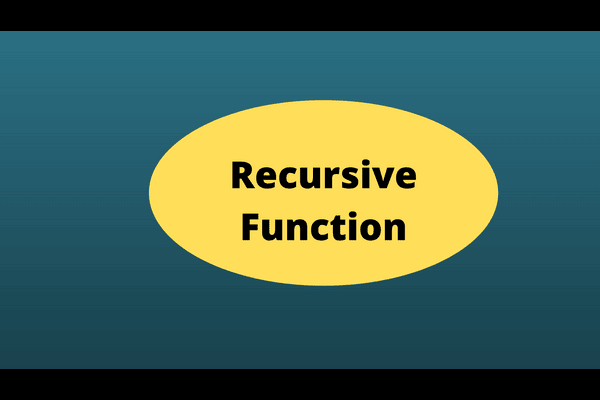### Bash Recursive Function How to Write it QuicklyHere's a simple bash recursive function. That tells how to use it. You can write these in JavaScript and other scripting languages. This specific example shows you how to write it in Bash shell.

## Recursive in the shell script

Calling the same function within it is called the Recursive function. How it works - we call itself and its contents.

Usually, the recursive functions go in the loop due to self-calling. Your code must have a condition that breaks the loop.

### Script logic

#!/bin/bash
for ((i = 1; i < 65; i++))
{ ((arr[i - 1] = i)) } i = 1;
key = 8

function linear_search {

echo "Element value: \${arr[\$i-1]}"
if ((arr[i - 1] == key)) then
echo "Linear search found \$key on attempt \$i" return 0 ==> it breaks the loop
else ((i++)) linear_search == > Calling th same script fi

linear_search == > Calling the same script

#### Output

Here is the dissection of output. Displayed the array's elements until match-condition occurs. In the end, a display statement tells at which point the condition is satisfied.Summary

• The For Loop's purpose is to get elements one by one
• The "arr" is a keyword for an Array in bash
• The i-1 is to calculate an index for the array

References